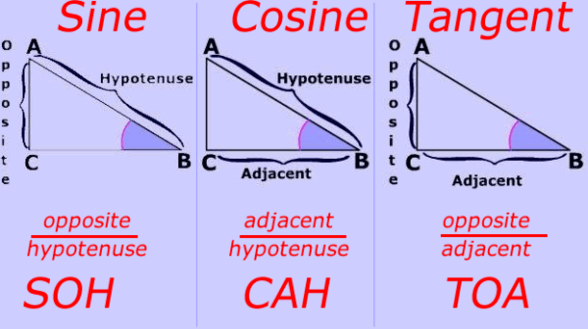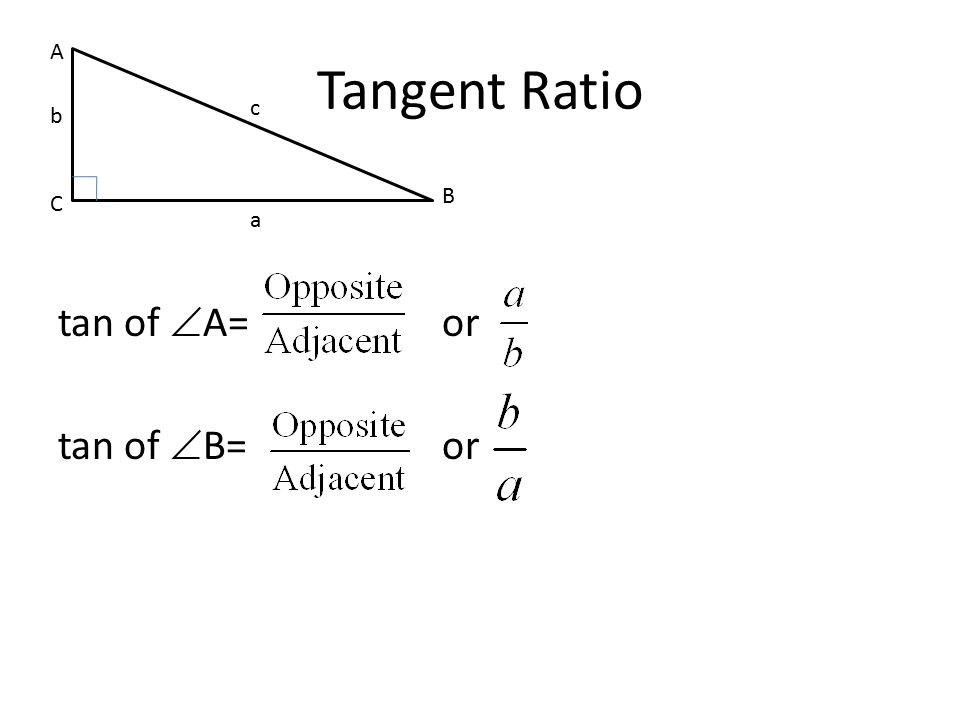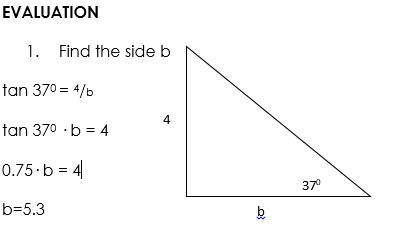# Tangent of an Angle

Welcome to Class !!

In today’s Mathematics class, We will be talking about Tangent of an Angle. We hope you enjoy the class!The tangent is one of the three basic trigonometric functions, the other two being sine and cosine. These functions are essential to the study of triangles and relate the angles of the triangle to its sides. The simplest definition of the tangent uses the ratios of the sides of a right triangle, and modern methods express this function as the sum of an infinite series. Tangents can be calculated directly when the lengths of the sides of the right triangle are known and can also be derived from other trigonometric functions.

Step 1

Identify and label the parts of a right triangle. The right angle will be at vertex C, and the side opposite it will be the hypotenuse h. The angle θ will be at vertex A, and the remaining vertex will be B. The side adjacent to angle θ will be side b and the side opposite angle θ will be side A. The two sides of a triangle that are not the hypotenuse are known as the legs of the triangle.

Step 2

Define the tangent. The tangent of an angle is defined as the ratio of the length of the side opposite the angle to the length of the side adjacent to the angle. In the case of the triangle in Step 1, tan θ = a/b.Step 3

Determine the tangent for a simple right triangle. For example, the legs of an isosceles right triangle are equal, so a/b = tan θ = 1. The angles are also equal so θ = 45 degrees. Therefore, tan 45 degrees = 1.

Step 4

Derive the tangent from the other trigonometric functions.

Since

then

The ratio called tangent (tan) of an acute angle in a right-angled triangle is defined as the ratio between the side opposite the angle and the side adjacent to the angle.

Example 1

Find the angle A

First

We need to use the inverse function for tan (i.e tan-1)to find the angle. This function is on the same key on the calculator as the tan function (shift tan).

We use the following sequence of commands:

shift    –     tan-1   0.75     = 37º

Try the following on your calculator to see the difference between tan and  tan-1:

angle → ratio                            ratio → angle

tan 370     =     0.75                   tan1 0.75    =  370We have come to the end of this class. We do hope you enjoyed the class?

Should you have any further question, feel free to ask in the comment section below and trust us to respond as soon as possible.

In our next class, we will be talking about Measures of Central Tendency. We are very much eager to meet you there.

Are you a Parent? Share your quick opinion and win free 2-month Premium Subscription

Don`t copy text!